Egwald Economics: Macroeconomics

by

Elmer G. Wiens

Egwald's popular web pages are provided without cost to users.
Please show your support by joining Egwald Web Services as a Facebook Fan:Follow Elmer Wiens on Twitter:Macroeconomics Theory, Testing, and Applications

The Basic Keynesian IS-LM Model

Closed Canadian Economy Version

 Closed Canadian Economy Version Macroeconomic Aggregates, Markets, and Agents. Macroeconomics attempts to understand the behaviour of the whole economy by analyzing the determination and interaction of such broad economic aggregates as national income and product, consumers' expenditures and savings, producers' output of products and producers' investment in capital, government revenues (taxes) and expenditures, exports and imports, the level and composition (by age, sex, and region) of employment, and the quantity of money in circulation. In the basic IS-LM model, the behaviour of the economic agents - consumers, producers (firms), and the government - is reconciled by the product and money markets. The product market balances the demand for product by consumers, firms, and the government with national income. The money market balances the demand for money by individuals (consumers) and firms with the supply of money provided by the government and the banks. The equilibrium the IS-LM model obtains is the demand equilibrium for the economy. All economic aggregates, including national income and the rate of interest adjust, so that the demand for product equals national income, and the demand for money equals the supply of money. Many important macroeconomics aggregates (variables), such as the levels of prices and wages, the volume of exports and imports, the pattern of foreign currency exchange rates, the stock of capital, and the wealth of consumers are left out of the basic IS-LM model of a closed economy. I also ignore the impact of the labour, bond, and stock markets on macroeconomics aggregates. Consequently, the equilibrium rate of interest and level of national income, plus the values of other macroeconomics aggregates produced by the IS-LM model, reflect only the demand side of the economy. Below, I specify the functions (equations) that describe the macroeconomics activities of the economic agents: consumers' demand for product, private firms' demand for product, and the government's collection of taxes, expenditures on product, and supply of money. I set the values of the parameters of the macroeconomics functions to reflect the average values of the macroeconomics aggregates of the Canadian economy during the years of 1998 - 2003. (Statistics Canada: Gross domestic product, income-based;   Gross domestic product, expenditure-based. Use the Add/Remove Icon to select years.)   I express all aggregates in billions of dollars.

 Consumers (Individuals). The Consumer Expenditure Function. The consumer expenditure function is a behavioural relation describing the demand for consumer goods by individuals. I hypothesize that the expenditures of consumers, c, is a function of disposable income, yd, and the rate of interest, r. Disposable national income equals national income less taxes paid to the government. c = c(yd, r) c = c1 + c2*yd - c3*r + c4*r2 c1, c2, c3, c4 >= 0 For the Canadian economy I use: c = 100 + 0.7*yd - 20*r + 0.5*r2 In the diagram below, I fixed (set) the rate of interest r at 3.35 yielding the demand for consumption expenditures c as a function of disposable income yd. Consumers' demand with the rate of interest r fixed is a linear function of yd. c = c(yd) = 38.61 + 0.7*yd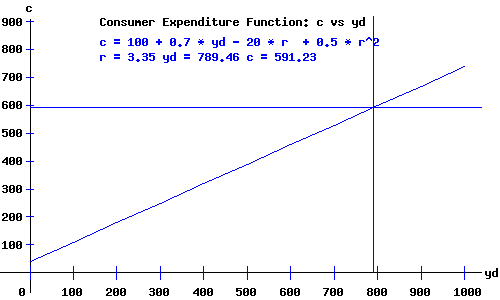Similarly, in the diagram below, I fixed disposable income at 789.46 yielding the demand for consumption expenditures c as a function of the rate of interest r. Consumers' demand with yd fixed is a quadratic function of r. c = c(r) = 652.62 - 20*r + 0.5*r2Producers (Firms). The Private Firms' Investment Function. The investment function is a behavioural relation describing the demand for investment goods (investment in capital) by private firms. I hypothesize that the investment demand of producers, i, is a function of national income, y, and the rate of interest, r. i = i(y, r) i = i1 + i2*y - i3*r + i4*r2 i1, i2, i3, i4 >= 0 For the Canadian economy I use: i = 100 + 0.2*y - 40*r + 1.5*r2 In the diagram below, I fixed the rate of interest r at 3.35 yielding the demand for investment goods i as a function of national income y. Producers' demand with the rate of interest r fixed is a linear function of y. i = i(y) = -17.17 + 0.2*y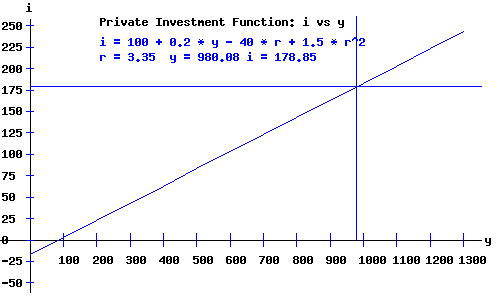Similarly, in the diagram below, I fixed national income at 980.08 yielding the demand for investment goods i as a function of the rate of interest r. Producers' demand with y fixed is a quadratic function of r. i = i(r) = 296.02 - 40*r + 1.5*r2Consumers and Producers. The Money Demand Function. The money demand function is a behavioural relation describing the demand for money by individuals and firms. Individuals hold money for transactions purposes, to buy real-goods and services, and as a store of wealth. As national income increases, individuals and firms need more money on hand to pay for the increase in their purchases of goods and services. I hypothesize that money demand, md, is an increasing function of national income, y. As interest rates increase, holding interest bearing securities (bonds) becomes increasingly more attractive than holding money. I hypothesize that money demand, md, is a decreasing function of the rate of interest, r. md = md(y, r) md = m1 + m2*y - m3*r + m4*r2 m1, m2, m3, m4 >= 0 For the Canadian economy I use: md = 75 + 0.23*y - 35*r + 1.5*r2 In the diagram below, I fixed the rate of interest r at 3.35 yielding the demand for money md as a function of disposable income y. Money demand with the rate of interest r fixed is a linear function of y. md = md(y) = -25.42 + 0.23*y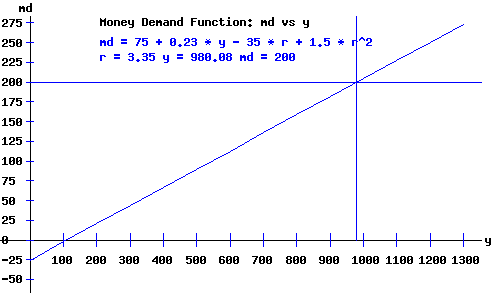Similarly, in the diagram below, I fixed national income at 980.08 yielding the demand for money md as a function of the rate of interest r. Demand for money with national income y fixed is a quadratic function of the rate of interest r. md = md(r) = 300.42 - 35*r + 1.5*r2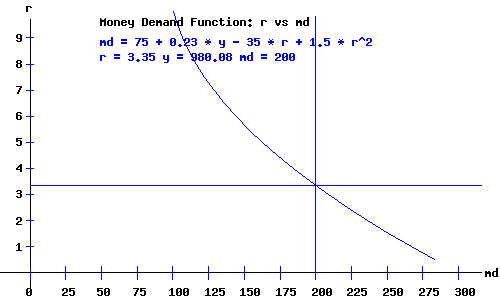The Government. Revenues and the Tax Function. The principal revenue sources for the federal government include income taxes, consumption taxes, social security contributions, and investment income. I hypothesize that value of government tax revenues, t, is a linear function of national income, y. t = t(y) t = -t1 + t2*y t1, t2 >= 0 For the Canadian economy I use: t = -25 + 0.22*y = -25 + 0.22 * 980.08 = 190.62.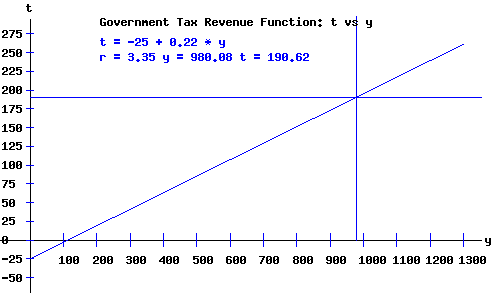Disposable Income Disposable national income, yd equals national income less taxes: yd = y - (-25 + 0.22*y) = 25 + 0.78*y = 25 + 0.78 * 980.08 = 789.46. Expenditures. The principal expenditures of the federal government include expenditures on social services, protection of persons and property, debt charges, resource conservation and industrial development. In this basic macroeconomic model, I fixed government expenditures g = 210 billion dollars.
 Money Supply. The Bank of Canada is Canada's Central Bank. It serves as the bank for the Government of Canada and Canada's chartered banks. The Bank of Canada controls the currency in circulation in Canada, and influences the money supply by manipulating interest rates (Summary of Key Monetary Policy Variables). For this basic IS-LM model, I define the money supply as currency outside of banks + personal chequing accounts + current accounts + a percentage of personal savings deposits and non-personal notice deposits. I fixed the money supply ms = 200 billion dollars.

 The Product (Commodity) Market Equilibrium. The commodity markets equilibrate the demand and supply for consumer goods and services, investment goods, and goods and services purchased by the government. I aggregate the value of these products into one generic good. The basic IS-LM model ignores the "supply side" of the economy - what quantities and types of products firms produce, and what factors of production (labour, capital services, and equipment and supplies) firms employ. I assume that the value of the supply of products produced will adjust to meet the value of the demand for products —> aggregate supply, AS = aggregate demand, AD. Furthermore, expenditures on products become payments compensating the factors (labour, capital, materials and supplies) that produced the output. Individuals, the ultimate owners of these factors of production, dispose of the income obtained on consumer expenditures, tax payments to the government, and savings. The product market equilibrium occurs at the level of national income where AD = AS, i.e., the level of income y where c + i + g = y. The diagram below depicts the equilibrium where c + i + g crosses the line (in yellow) with a 45 degree angle with the y-axis.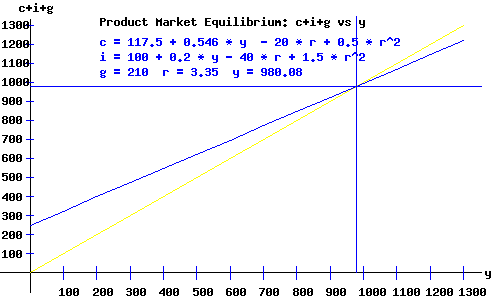The c + i + g curve as a function of r and y is: c + i + g = 427.5 + 0.746*y - 60*r + 2*r2 The IS Curve. Because the demand for consumer goods and the demand for producer goods vary inversely with the rate of interest r, the c + i + g curve as a function of national income y shifts upward as the rate of interest r decreases. Consequently, a lower rate of interest requires a higher level of national income for equilibrium in the commodity market. The locus of interest rates and national incomes implicit for equilibrium in the commodity (product) market, an inverse relation between the rate of interest and national income is called the IS curve. Click to pop a new window with a graphical derivation of the IS curve. The IS curve, y as a function of the rate of interest r, is: y = 1683.07 - 236.22 * r + 7.87 * r2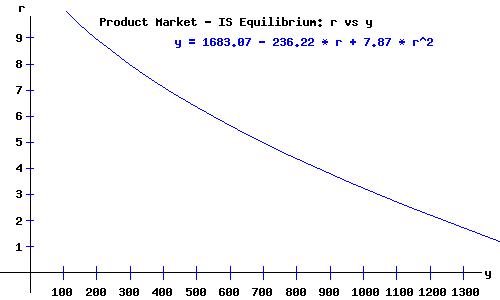The Money Market Equilibrium. The Bank of Canada's key overnight policy interest rate influences the chartered banks' prime interest rates (and the availability of money to lend to their best customers). Interest rates adjust throughout the economy affecting investment and consumption demand, and thereby the equilibrium national income of the IS-LM model of the demand side of the economy, until the demand for money equals the supply of money. In the basic IS-LM model, I assume the government sets the supply of money at a specific level. The diagram below depicts the equilibrium where the demand for money curve intersects the vertical supply of money line.The LM Curve. The demand for money is an increasing function of national income y. Therefore, the demand for money curve, as a function of the rate of interest, shifts upwards with an increase in national income. Consequently, a higher level of national income requires a higher rate of interest for equilibrium in the money market. The locus of interest rates and national incomes implicit for equilibrium in the money market, a direct relation between the rate of interest and national income is called the LM curve. Click to pop a new window with a graphical derivation of the LM curve. The LM curve, y as a function of the rate of interest r, is: y = 543.48 + 152.17 * r - 6.52 * r2The IS-LM Demand Equilibrium.

The basic IS-LM economy is in equilibrium when national income, y, and the rate of interest, r, are at levels consistent with equilibrium in both the product and money markets.

Economy equilibrium occurs where the IS curve and the LM curve intersect.

As a review, I list the system of equations for the IS-LM model. Solving these equations simultaneously, I obtain the values of the endogenous macroeconomics variables of the model: the amount of output and national income, y, and the rate of interest, r.

 Product Market - IS Demand Equilibrium Consumers c = 100 + 0.7*yd - 20*r + 0.5*r2 Producers i = 100 + 0.2*y - 40*r + 1.5*r2 Government Expenditures g = 210 Government Revenues t = -25 + 0.22*y Consumer Disposable Income yd = y - (-25 + 0.22*y) IS Curve c + i + g = y Money Market - LM Demand Equilibrium Consumers & Producers md = 75 + 0.23*y - 35*r + 1.5*r2 Money Supply ms = 200 ; LM Curve md = ms

For this basic model of the Canadian economy, the equilibrium obtains where

y = 980.08,     r = 3.35

The diagram below depicts the IS-LM economy equilibrium.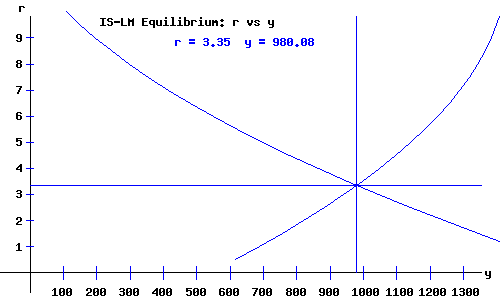Economy Equilibrium Macroeconomic Variables and Aggregates

 National Income: y 980.08 Rate of Interest: r 3.35 Disposable National Income: yd 789.46 Consumer Expenditures: c 591.23 Firm Investments: i 178.85 Government Expenditures: g 210 Government Revenue: t 190.62 Money Supply: ms 200 Consumer Savings: s = yd - c 198.23

The IS-LM Model's results versus the National Economic and Financial Accounts.

Comparing the model's results with the Statistics for the Canadian Economy reveals the above IS-LM Model's limits and inadequacies. For example, the Federal Government (Federal government revenue and expenditures), and the Canadian governments (Consolidated government revenue and expenditures) operated at a surplus during the years 1999 to 2003. Many other factors inhibit the realism of the IS-LM Model. (Use the Add/Remvoe Data Icon on ths Statistics Canada web pages if necessary).

The Comparative Statics Of The Basic Keynesian IS-LM Model web page permits you to adjust the parameters of the IS-LM Model. See if you can obtain more realistic results (like running a government surplus without reducing national income).

Works Cited and Consulted

• Akerlof, George A. "The Missing Motivation in Macroeconomics." Presidential Address, AEA, January, 2007.
• Branson, William H. and James M. Litvack. Macroeconomics. New York: Harper, 1976.
• Crouch, Robert L. Macroeconomics. New York: Harcourt, 1972.
• Darby, Michael. Macroeconomics. New York: McGraw-Hill, 1976.
• Dornbusch, Rudiger. Open Economy Macroeconomics. New York: Basic, 1980.
• Dornbusch, Rudiger, Stanley Fischer, and Gordon Sparks. Macroeconomics, 1st. Canadian Edition. Toronto: McGraw-Hill, 1982.
• Laidler, David E. W. The Demand For Money: Theories and Evidence. Scranton, Penn.: International Textbook, 1969.
• Parkin, Michael, and Robin Bade. Macroeconomics. 4th ed. Toronto: Addison Wesley, 2000.Copyright © Elmer G. Wiens:   EgwaldWeb ServicesAll Rights Reserved.    Inquiries